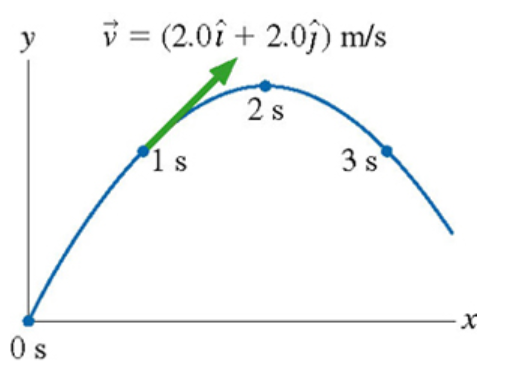# Problem: A physics student on Planet Exidor throws a ball, and it follows the parabolic trajectory shown in the figure. The ball's position is shown at 1s intervals until t=3s. At t=1s , the ball's velocity is v = (2.0 i + 2.0 j) m/s.Determine the ball's velocity at t = 2s

###### FREE Expert Solution

At t = 0s, the ball is at the maximum height. At maximum height, vy = 0 m/s.

91% (477 ratings)###### Problem Details

A physics student on Planet Exidor throws a ball, and it follows the parabolic trajectory shown in the figure. The ball's position is shown at 1s intervals until t=3s. At t=1s , the ball's velocity is v = (2.0 i + 2.0 j) m/s.

Determine the ball's velocity at t = 2s